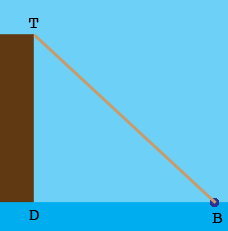SEARCH HOMEMath Central Quandaries & Queries$D$ to $B$ Question from Tracey: A boat is tied with a rope to a dock that is 16 feet tall. Along, the water the boat is 17 feet from the dock. How long is the rope connecting the boat to the dock? Let c represent the length of the rope.?Hi Tracey,

In my diagram $T$ is the top of the dock, $D$ is the point where the dock meets the water and $B$ is the boat.The distance from $T$ to $D$ is 16 feet and the distance from $D$ to $B$ is 17 feet and the distance from $T$ to $B$ is the length of the rope. The triangle $TDB$ is a right triangle. Pythagoras can tell you the length of the rope.

Penny* Registered trade mark of Imperial Oil Limited. Used under license.Math Central is supported by the University of Regina and the Imperial Oil Foundation.